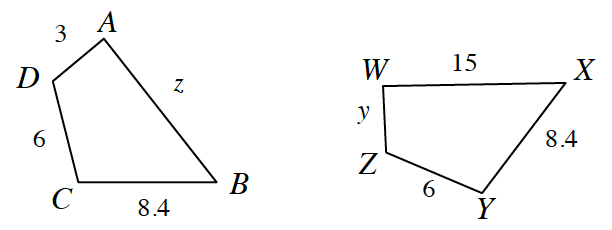### Home > GB8I > Chapter 8 Unit 9 > Lesson INT1: 8.1.1 > Problem8-18

8-18.

Use tracing paper and a series of rigid transformations to determine if these two polygons are congruent. If possible, find the lengths of $z$ and $y$.What two transformations are needed to move $ABCD$ onto $WXYZ$?

To find $z$ and $y$, divide or multiply by the zoom factor.

$y=3$